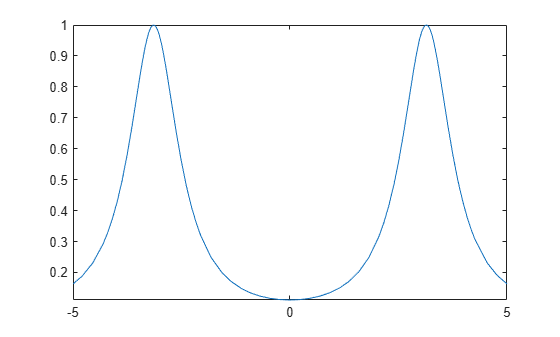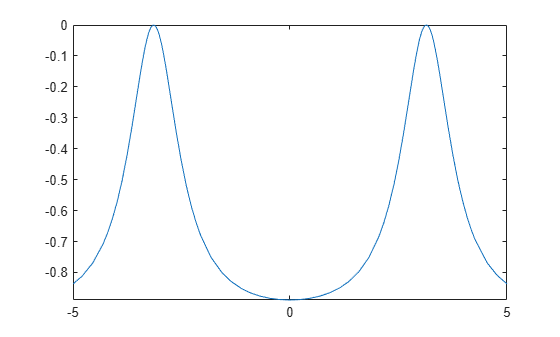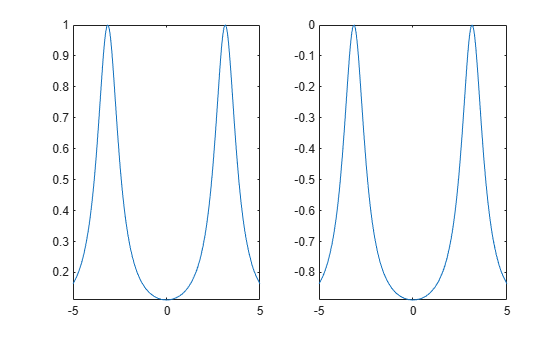Documentation

## Learn Calculus in the Live Editor

Learn calculus and applied mathematics using the Symbolic Math Toolbox™. The example shows introductory functions `fplot` and `diff`.

To manipulate a symbolic variable, create an object of type `syms`.

`syms x`

Once a symbolic variable is defined, you can build and visualize functions with `fplot`.

`f(x) = 1/(5+4*cos(x))`
```f(x) =  $\frac{1}{4 \mathrm{cos}\left(x\right)+5}$```
`fplot(f)`Evaluate the function at $x=\pi /2$ using math notation.

`f(pi/2)`
```ans =  $\frac{1}{5}$```

Many functions can work with symbolic variables. For example, `diff` differentiates a function.

`f1 = diff(f) `
```f1(x) =  $\frac{4 \mathrm{sin}\left(x\right)}{{\left(4 \mathrm{cos}\left(x\right)+5\right)}^{2}}$```
`fplot(f1) ``diff` can also find the ${N}^{th}$ derivative. Here is the second derivative.

`f2 = diff(f,2) `
```f2(x) =  $\frac{4 \mathrm{cos}\left(x\right)}{{\left(4 \mathrm{cos}\left(x\right)+5\right)}^{2}}+\frac{32 {\mathrm{sin}\left(x\right)}^{2}}{{\left(4 \mathrm{cos}\left(x\right)+5\right)}^{3}}$```
`fplot(f2) ``int` integrates functions of symbolic variables. The following is an attempt to retrieve the original function by integrating the second derivative twice.

`g = int(int(f2)) `
```g(x) =  $-\frac{8}{{\mathrm{tan}\left(\frac{x}{2}\right)}^{2}+9}$```
`fplot(g)`At first glance, the plots for $f$ and $g$ look the same. Look carefully, however, at their formulas and their ranges on the y-axis.

```subplot(1,2,1) fplot(f) subplot(1,2,2) fplot(g)```$e$ is the difference between $f$ and $g$. It has a complicated formula, but its graph looks like a constant.

`e = f - g `
```e(x) =  $\frac{8}{{\mathrm{tan}\left(\frac{x}{2}\right)}^{2}+9}+\frac{1}{4 \mathrm{cos}\left(x\right)+5}$```

To show that the difference really is a constant, simplify the equation. This confirms that the difference between them really is a constant.

`e = simplify(e) `
`e(x) = $1$`

#### Mathematical Modeling with Symbolic Math Toolbox

Get examples and videos